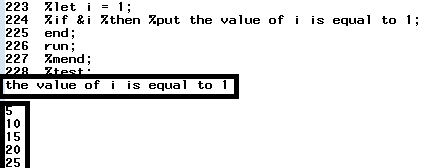# When to use IF and %IF in SAS Macros

Most of the programmers who are new to SAS macro programming are confused between the IF condition and %IF in SAS Macros. The usage of DO END and %DO %END in macro programming also creates confusion. This tutorial describes the difference with examples which would help to understand the practical usage of these techniques.

When to use IF and %IF in SAS Macros

1. IF statement cannot be used outside data step whereas %IF can be used outside and inside data step but within the macro.

Example 1 :

In the following program, we are telling SAS to check the value if it is greater than 10 and then run procedure dependending on the conditional statement.

%IF works to run procedures -
%macro temp(N=);
%if &N. > 10 %then %do;
proc means data = sashelp.class MEAN;
var age;
run;
%end;
%else %put better luck next time;
%mend;
%temp(N=19);
IF Statement does not work to run procedure.
%macro temp2(N=);
data _null_;
if &N. > 10 then do;
proc means data = sashelp.class MEAN;
var age;
run;
end;
else put "the value is less than or equal to 10";
run;
%mend;
%temp2(N=11);

2. The %IF can only be used inside of a macro. In other words, you cannot use it in a program without it in a macro definition (%macro yourmacro; %mend;)

The following program does not work as the %IF is not valid outside macro.
%let N = 12;
%if &N. > 10 %then %do;
proc means data = sashelp.class MEAN;
var age;
run;
%end;
%else %put better luck next time;

3. .SAS Macro will be executed first and once completed, data step statements will be executed.

%macro test;
data temp;
do j =1 to 5;
N = j *5;
put N;
%let i = 1;
%if &i %then %put the value of i is equal to 1;
end;
run;
%mend;
%test;%IF vs IF statement
First Step - SAS checks for macro statements/macro variables and executing them. In this case, it sets i is equal to 1, then writes a log " the value of i is equal to 1". Once this process is completed, it checks DO LOOP and run iterations and multiply each iteration value by 5 and prints to the log.

When either of DO END or %DO %END can be used

If you're generating code to automate repetitive task within a data step, and you can use either %do-%end or do-end.

In the following program, we are generating 5 values starting from 5 and ends with 25 with the difference of 5 between values.

Method I : DO END
data temp;
do j =1 to 5;
N = j *5;
output;
end;
drop j;
run;

Method II : %DO %END
%macro test;
data temp;
%do j = 1 %to 5;
N = &j. *5;
output;
%end;
run;
%mend;
%test;
Related Posts
Share1.